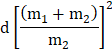# Two pendulums each of lengthare initially situated as shown in fig. The first pendulum is released and strikes the second. Assume that the collision is completely inelastic and neglect the mass of the string and any frictional effects. How high does the centre of mass rise after the collision?a)b)c)d)## Question ID - 100173 :- Two pendulums each of lengthare initially situated as shown in fig. The first pendulum is released and strikes the second. Assume that the collision is completely inelastic and neglect the mass of the string and any frictional effects. How high does the centre of mass rise after the collision?a)b)c)d)3537

(a)

Applying the law of conservation of momentum,(i)

Whereis the velocity with whichcollides withTherefore,Now,let the centre of mass rise through a heightafter collision. In this case, the kinetic energy ofsystem is converted into potential energy at maximum heightNext Question :
 The size of image formed by a concave mirror is same as the size of object. The position of the object will be (a) at F (b) between F and C (c) at C (d) between C and infinity